# Test: Even And Odd Integers- 1

## 10 Questions MCQ Test Quantitative Aptitude for GMAT | Test: Even And Odd Integers- 1

Description
Attempt Test: Even And Odd Integers- 1 | 10 questions in 20 minutes | Mock test for GMAT preparation | Free important questions MCQ to study Quantitative Aptitude for GMAT for GMAT Exam | Download free PDF with solutions
QUESTION: 1

### Set P consists of the first n positive multiples of 3 and set Q consists of the first m positive multiples of 5. The sum of all the numbers in set P is equal to R and the sum of all the numbers in set Q is equal to S. If m and n are positive integers, is the difference between R and S odd? (1) m is odd and n is even (2) m can be expressed in the form of 4x +3 and n can be expressed in the form of 2x, where x is a positive integer

Solution:

Steps 1 & 2: Understand Question and Draw Inferences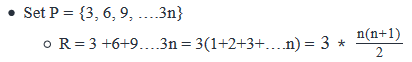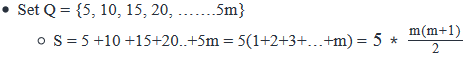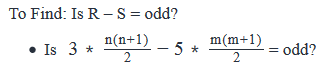• Difference of two integers will be odd, only if one of the numbers is odd.
• Let’s see when R and S will be odd:
• The even-odd nature of R will depend on the values of n and n + 1. Since n and n+ 1 are consecutive integers, only one of them can be even.
• Now, in the expression of R as n(n+1) is divided by 2, for R to be even, either of n or n + 1 should be a multiple of 4, i.e. they should be of the form 4k, where k is a positive integer. Hence, following cases arise:
• n is of the form 4k, i.e. n = 4k OR
• n+1 is of the form 4k, i.e. n + 1 = 4k
• So, n = 4k – 1 = 4(k -1) + 4 -3 = 4(k-1) + 3
• So, we can say that R will be even if n is of the form 4k or 4k + 3. For all the other cases, R will be odd
• Since the expression of S is similar to R, we can say that S will be even if m is of the form 4k or 4k + 3
• So, we need to look for values of m and n in the statements.

Step 3: Analyze Statement 1 independently

(1) m is odd and n is even

• As we do not know if m or n is of the form of 4k or 4k + 3, we cannot say, if R and S are odd or even.

Step 4: Analyze Statement 2 independently

(2) m can be expressed in the form of 4x +3 and n can be expressed in the form of 2x, where x is a positive integer

• As m can be expressed in the form of 4x + 3, S will be even.
• As n can be expressed in the form of 2x, n may be expressed in the form of 4x or may not be expressed in the form of 4x.
• So, R may be odd or R may be even.
• If R is odd,  R – S = odd
• If R is even, R – S = even.
• Hence, we cannot say for sure if R – S is odd.

Step 5: Analyze Both Statements Together (if needed)

(1) From statement-1, we know that  is odd and n is even

(2) From statement-2, we know that R may be even or odd and S is even

• Combining both the statements does not tell us anything about the even-odd nature of R, hence we cannot say for sure if R – S = odd.

QUESTION: 2

### If  a,b and c are three positive integers such that at least one of them is odd, which of the following statements must be true?

Solution:

Given

• a, b, c are integers > 0
• At least one of a, b, c is odd. So possible cases can be:
• Only one of (a, b, c) is odd
• Two of (a, b, c) are odd
• All (a, b, c, ) are odd

To Find: the option that must be true (for all possible values of a,b and c)

Approach

1. As the options involve the combination of sum of a, b  and c, we will need to evaluate the options to see if they are always true for the possible combinations of the numbers (a, b, c) being odd or even

Working Out

1. a+b+c is odd
1. Sum of 3 integers will be odd only if:
1. One of the integers is odd
2. OR all three integers are odd
2. However, they may be a case where two of integers are odd and one is even. In such a case, a + b + c = even.
3. So, option-I is not always true.
2. a+b+c and abc have the same even-odd nature
1. Consider the case, where abc is even. This is possible if:
1. 1 of a, b, c is even→ In this case, a+ b + c  = even
2. 2 of a, b, c, is even→ In this case, a+ b + c = odd
3. All of a, b, c, are even→ In this case a + b + c = even
2. As we can see from above that the even-odd nature of a+b+c and abc are not always the same, this option is not always true
3. 100a + 10b + c is odd
1. The even-odd nature of 100a +10b + c will depend on even-odd nature of c, because 100a and 10b are always even.
1. If c is odd, 100a +10b +c is odd
2. If c is even, 100a +10b + c is even
2. So, option-III is not always true
4. If b/2 and c/2 are integers,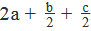is even
1. Since b/2 and c/2 are integers, b and c must be even
2. As 2a must be even, b/2+c/2  should be even forto be even.
1. Sum of two integers can be even, only if both are even or both are odd. Following cases can arise:
1. If b and c are multiples of 4→b/2 and c/2 will be even
1. Hence ​​​will be even
2. If either of b and c are not multiples of 4→ In this case only one of b/2 or c/2  will be even and the other will be odd.

Hence b/2+c/2 will be odd.

• Hencewill be odd

So, option-IV is not always true.

As none of the options are true, the correct answer will be option E

QUESTION: 3

### If n is an integer, the number of integers between, but not including, n and 3n cannot be I. 13 II. 14 III. 15

Solution:

Given:

• Integer n

To find: Which of the following CANNOT be the number of integers between, but not including, n and 3n: {13, 14, 15}

Approach:

1. To answer this question, we’ll work out a constraint on the number of integers between, but not including, n and 3n
2. Then, we’ll use this constraint to check which of the 3 values is not possible.

Working Out:

• Number of integers between 3n and n = 3n – n = 2n
• But this count includes 3n itself.
• For example, say n = 1. Then, if you do 3 – 1, you get 2. That is, 2 integers lie between 1 and 3. This count includes 3.
• So, the Number of integers between, but not including, 3n and n = 2n – 1

• Thus, the number of integers between and not including n and 3n can only be an odd number.
• So, this number cannot be 14.

Looking at the answer choices, we see that the correct answer is Option B

QUESTION: 4

If z is an integer greater than 1, is z even?

(1) 2z is not a factor of 8

(2) 3z/4  is a factor of 6

Solution:

Steps 1 & 2: Understand Question and Draw Inferences

Given: Integer z > 1

To find: Is z even?

Step 3: Analyze Statement 1 independently

• 2z is not a factor of 8
• Factors of 8: {1, 2, 4, 8}
• This means, 2z is not equal to 1, 2, 4, 8
• z is not equal to ½ or 1(anyways not possible since z is an integer greater than 1)
• And, z is not 2 or 4
• But z can be equal to 8 or 10 or 12 etc.
• That is, z can still be an even number.

Thus, Statement 1 is not sufficient to answer the question

Step 4: Analyze Statement 2 independently

• 3z/4  is a factor of 6
• Factors of 6: {1, 2, 3, 6}
• This means 3z/4 is an integer and is equal to one of these 4 numbers.
• Since 3z/4 is an integer, z must be divisible by 4.
• Therefore, z is indeed even.

Statement 2 is sufficient to answer the question

Step 5: Analyze Both Statements Together (if needed)

Since we’ve already arrived at a unique answer in Step 4, this step is not required

QUESTION: 5

Set A consists of a set of n consecutive integers. Is the sum of all the integers in set A even?

(1) If -5 is added to set A, the set would become symmetric about 0

(2) If the largest integer of set A is removed, the sum of the remaining integers is even

Solution:

Steps 1 & 2: Understand Question and Draw Inferences

• Let the smallest integer be in set A be a. So, the other integers in set A will be a+1, a+2….a+n-1
• Sum of all integers in set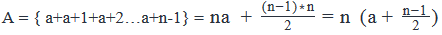•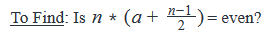Step 3: Analyze Statement 1 independently

(1) If -5 is added to set A, the set would become symmetric about 0

• As the set becomes symmetric about 0, that would mean that there will be (upon the inclusion of -5 in set A) an equal number of terms on either side of 0 with the same magnitude (but opposite signs).
• Let’s call this new set as A’
• So, the mean of (Set A’) = 0
• So, the sum of (All the integers in set A’) = 0
•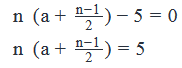• Hence, the sum of all the integers in set A is odd.

Step 4: Analyze Statement 2 independently

(2) If the largest integer of set A is removed, the sum of the remaining integers is even.

• The largest integer of Set A = a +n -1.
• So, (Sum of all the terms in set A – largest integer of set A) = even
• Let’s think of the possible ways in which difference of two integers is even:
• Case-I: even – even = even
• So, if the largest integer removed is even, the sum of all the integers in set A would be even.
• Hence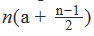will be even
• Case-II: odd – odd = even
• So, if the largest integer removed is odd, the sum of all the integers in set A would be odd.
• Hence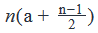will be odd
• As we do not have a unique answer, the statement is insufficient to answer.

Step 5: Analyze Both Statements Together (if needed)

• As we have a unique answer from step-3, this step is not required.

QUESTION: 6

If A and B are integers, is the product AB even?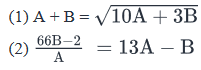Solution:

Steps 1 & 2: Understand Question and Draw Inferences

Given: Integers A and B

To find: Is the product AB even?

• The answer will be YES if at least one of A and B is even

Step 3: Analyze Statement 1 independently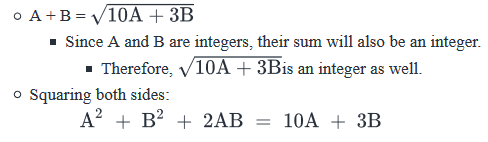• In the above equation, 2AB and 10A will always be even. So, we can write: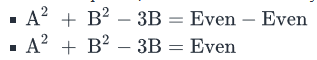Case 1: B is even

• Then, B2 and 3B will both be even
• The difference of B2 – 3B will be Even as well
• A2 + Even  = Even
• This is possible only if A is even
• Since both A and B are even, the product AB will be even

Case 2: B is odd

• Then, B2 and 3B will both be odd
• The difference of B2 – 3B will be Even
• A2 + Even  = Even
• This is possible only if A is even
• Since A is even, the product AB will be even

This means, the product AB is definitely even.

Statement 1 is sufficient to answer the question

Step 4: Analyze Statement 2 independently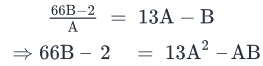• The left hand side of this equation is the difference of 2 even terms. So, the left hand side is Even
• This means, the Right hand side of the equation must be Even as well. So, we can write:

13A2 - AB =Even

• Case 1: B is even
• Then, AB is even
• Since the above equation holds true, 13A2 must be Even as well.
• This means, A is even as well (since 13A2 has the same even-odd nature as A)
• Since both A and B are even, the product AB is Even.

• Case 2: B is odd
• Then, AB has the same even-odd nature as A
• Also, 13A2 will have the same even-odd nature as A
• Thus, the left hand side of the equation has the difference of two terms which are either both odd or both even.
• We know that Odd – Odd = Even and Even- Even = Even
• Thus, the above equation will be satisfied whether A is even or odd
• If A is even, then the product AB will be even
• But if A is odd, then the product AB will be odd
• Thus, we see that the product AB may be either even or odd. Statement 2 is not sufficient to determine a definite answer about the even-odd nature of AB.

Step 5: Analyze Both Statements Together (if needed)

• Since we’ve already arrived at a unique answer in Step 3, this step is not required
QUESTION: 7

If A and B are positive integers, is A – B even?

1. The product of A and B is even

2. A + B is odd

Solution:

Steps 1 & 2: Understand Question and Draw Inferences

Given: Integers A, B > 0

To find: Is A – B even?

• The answer will be YES if A and B have the same even-odd nature, i.e. A and B are both even or both odd

Step 3: Analyze Statement 1 independently

Statement 1 says that ‘The product of A and B is even’

• This means, that one number out of A and B is definitely even
• The other number may be even or odd
• So, A – B may be:
• Either Even – Odd = Odd
• Or Even – Even = Even
• Thus, Statement 1 is not sufficient to give a unique Yes/ No answer to the question

Step 4: Analyze Statement 2 independently

Statement 2 says that ‘A + B is odd’

• For A + B to be odd, one of A or B has to be even and the other odd.
• So, Even – Odd = Odd OR Odd – Even = Odd
• Hence A – B will be odd.
• Therefore, Statement 2 is sufficient to answer the question

Step 5: Analyze Both Statements Together (if needed)

Since we’ve already arrived at a unique answer in Step 4, this step is not required

QUESTION: 8

If P and Q are positive integers, then is (P+2)(Q-1) an even number?

(1) p/3Q is an even integer

(2)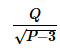is a positive odd integer

Solution:

Steps 1 & 2: Understand Question and Draw Inferences

Given: Integers P, Q > 0

To find:  Is (P+2)(Q-1) even?

• The answer is YES if
• At least one out of P + 2 and Q – 1 is even
• P + 2 is even if P is even
• Q – 1 is even if Q is odd
• So, the answer is YES if
• Either P is Even
• Or Q is odd, or both

Step 3: Analyze Statement 1 independently

• P/3Q  is an even integer.
• This means, P = (3Q)(2n) where n is a positive integer
• So, P is an even number
• Therefore, the answer is YES

Thus, Statement 1 is sufficient to answer the question

Step 4: Analyze Statement 2 independently

•is an odd integer
• So, we can write: Q=  (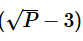) (2m+1), where m is an integer
• Since Q and 2m + 1 are both integers,(must be an integer as well
• Therefore,√P is an integer
• Implies, that P is a perfect square
•  √P has the same even-odd nature as P

• Case 1: P is even
• We’ve already determined in Steps 1 and 2 that if P is even, the answer to the question is YES

• Case 2: P is odd
• Q = (Odd - Odd)(Odd)
• Q = Even * Odd
• Q = Even
• Thus, Statement 2 doesn’t lead us to a unique answer to the question.

Therefore, Statement 2 is not sufficient.

Step 5: Analyze Both Statements Together (if needed)

Since we’ve already arrived at a unique answer in Step 3, this step is not required

QUESTION: 9

There are N students in a class. When the students are distributed into groups that contain 4A number of students each, 3 students are left without a group. When the students are distributed into groups that contain A/3 number of students each, no students are left without a group. Which of the following statements is correct?

I. If the students are distributed into groups that contain A+ 1 students each, the number of students that are left without a group can be 2

II. If the students are distributed into groups that contain 3 students each, no students are left without a group

III. If the students are distributed into groups that contain 12 students each, 9 students are left without a group

Solution:

Given:

• N = (4A)x + 3, where quotient x is an integer
• N=(A/3)k
• , where k is an integer
• Since N denotes the number of students, N must be a positive integer

To find: Which of the 3 statements is/are correct?

Approach:

1. To answer the question, we first need to get an idea about the values of N and A
2. Then, we’ll evaluate the 3 statements one by one. A statement will be a must be true statement only if it holds true for all possible values of N and A

Working Out:

• Drawing inferences about N and A
• We’re given that N is of the form (4A)x + 3
• Now, the term (4A)x will be even for all values of A and x
• So, N is of the form Even + Odd
• So, N is odd
• Also, in the second piece of given information, we’re told that each student group contains A/3 students
• Since number of students cannot be in fractions, this means A must be a multiple of 3
• Also, N=(A/3)k
• We’ve inferred above that N is odd
• So, the product of integers (A/3)
•  and k is odd
• This is possible only if (A/3)
•  and k themselves are odd
• So, (A/3)  is odd
• Thus, A = (Odd number 3)*(Another odd integer)
• So, A is odd as well
• Thus, combining our above inferences about A, we can say that A is an odd multiple of 3.
• So, we can write: A = 3(2B+1) = 6B + 3, where B is an integer

• Evaluating Statement I
• If the students are distributed into groups that contain A + 1 students each, the number of students that are left without a group can be 2
• A + 1 = (6B+3) + 1 = 6B + 4 = Even number
• Let the number of groups that contain A + 1 students be G.
• (Odd Number N) = (Even Number A+1)*(G) + Remainder
• So, (Odd Integer) = Even Integer + Remainder
• So, Remainder = Odd Integer minus Even Integer
• So, the remainder when N is divided by A +1 will be an odd integer.
• Therefore, the remainder cannot be 2
• So, Statement I is not correct

• Evaluating Statement II
• If the students are distributed into groups that contain 3 students each, no students are left without a group
• To answer this question, we need to evaluate if N is divisible by 3 or not
• If it is, then Statement II is indeed a must be true statement.
• Now, we’ve analyzed above that:
• N = (4A)x + 3
• And, A = 6B+3
• So, we can write: N = 4(6B+3)x + 3
• = 4*3(2B+1)x + 3
• =3{4(2B+1)x + 1}
• Thus, we see that N is completely divisible by 3
• So, Statement II is correct

• Evaluating Statement III
• If the students are distributed into groups that contain 12 students each, 9 students are left without a group
• To evaluate this statement, we need to determine if the remainder when N is divided by 12 is 9
• If it is, then this is a must be true statement.
• In our analysis of Statement II above, we’ve inferred that:
• N = 3{4(2B+1)x + 1}
• Simplifying this: N = 12(2B+1)x + 3
• So, when N is divided by 12, the first term in the above expression: 12(2B+1)x will be completely divisible by 12
• So, the remainder when N is divided by 12 will be 3
• Therefore, Statement III is incorrect
• From our analysis above, we’ve concluded that only Statement II is always correct.

Looking at the answer choices, we see that the correct answer is Option B

QUESTION: 10

Which one of the following is even?

Solution:

Step 1:

5 × 7 = 35
1 × 3 = 3
9 × 5 = 45

Step 2:
Only 3 × 4 = 12 is even. Rest are odd.Use Code STAYHOME200 and get INR 200 additional OFF Use Coupon Code